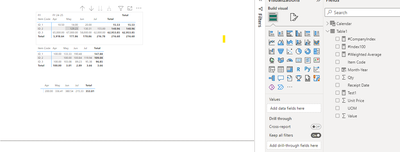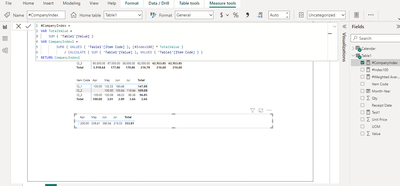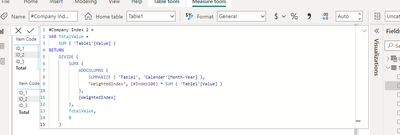cancel
Showing results for
Did you mean:

Fabric is Generally Available. Browse Fabric Presentations. Work towards your Fabric certification with the Cloud Skills Challenge.Frequent Visitor

## Indexing Using DAX

Hi Everyone!

I'm facing a challenge to create a company-wide Index using DAX in PowerBi.

Please refer below as my Purchase data.

 Item Code Receipt Date Unit Price Qty Value UOM ID_1 01-Apr-24 10 100 1000 KG ID_1 02-Apr-24 11 100 1100 KG ID_3 05-Apr-24 65000 20 1300000 TON ID_2 02-May-24 120 10000 1200000 ECH ID_1 05-May-24 14 220 3080 KG ID_2 10-May-24 160 3000 480000 ECH ID_3 18-May-24 67000 10 670000 TON ID_2 06-Jun-24 130 15000 1950000 ECH ID_1 07-Jun-24 20 300 6000 KG ID_2 09-Jun-24 135 1000 135000 ECH ID_2 12-Jul-24 155 23000 3565000 ECH ID_3 21-Jul-24 62000 23 1426000 TON

The output that is expected would look be Weighted Average of the Index that would be calculated.

Step 1: Weighted Average Matrix. (Simple Weighted Average of an Item for that month)

 Apr-24 May-24 Jun-24 Jul-24 ID_1 10.5 14 20 ID_2 129.231 130.3125 155 ID_3 65000 67000 620

Step 2: Index of that Weighted Average. (Using base as 100, we calculate the Index100. Challenge is to make the first nonblank month as 100)

 Apr-24 May-24 Jun-24 Jul-24 ID_1 100 133.3333 190.4762 ID_2 100 100.8369 119.9403 ID_3 100 103.0769 95.38462

Step 3: Weighted Average of the Index (Now the last part to calculate the index of the entire company. Taking weights as the sum of value for that paticular month and item, we calculate Weighted Average) i.e. SUMX(Index*Value)/SUM(Value).

(For e.g. April-24 would be =((100*2100+100*1300000)/(2100+1300000)
and   May-24 would be =((133.33*3080+100*1680000+103.0769*670000)/(3080+1680000+670000) and so on...

 Apr-24 May-24 Jun-24 Jul-24 ID_Index 100 100.9197 101.0941 112.9244

I'm facing difficulty when there is no entry for a paticular month, then the values become infinity.

Thanks,
Best Regards.

4 REPLIES 4Memorable Member

To achieve the desired result of calculating a company-wide index in Power BI using DAX, you can follow these steps. The key challenge is handling cases where there is no entry for a particular month, which can result in division by zero errors. To overcome this challenge, you can use conditional logic to check for valid data before performing calculations.

Here's a step-by-step approach:

Step 1: Weighted Average Matrix

To calculate the weighted average matrix for each item and month, you can use DAX measures like this:

WeightedAverage = VAR TotalValue = SUM('PurchaseData'[Value]) RETURN SUMX('PurchaseData', [Unit Price] * [Qty] / TotalValue )

Create one measure like this for each month. These measures will calculate the weighted average for each item and month.

Step 2: Index of Weighted Average

To calculate the index based on the weighted averages with the first non-blank month as 100, you can use the following DAX measure:

Index100 = VAR FirstNonBlankMonth = CALCULATE( MIN('Calendar'[Month]), FILTER( ALL('Calendar'), [WeightedAverage] > 0 ) ) RETURN IF(ISBLANK([WeightedAverage]), BLANK(), [WeightedAverage] / CALCULATE([WeightedAverage], 'Calendar'[Month] = FirstNonBlankMonth) )

This measure calculates the index based on the weighted average, and it checks for a non-blank weighted average to avoid division by zero.

Step 3: Weighted Average of the Index

To calculate the company-wide weighted average of the index, you can use this DAX measure:

CompanyIndex = VAR TotalValue = SUM('PurchaseData'[Value]) RETURN SUMX(VALUES('PurchaseData'[Item Code]), [Index100] * [Value] / CALCULATE(SUM('PurchaseData'[Value]), VALUES('PurchaseData'[Item Code])) )

This measure calculates the weighted average of the index for the entire company while considering valid data points. It divides the sum of the product of index and value by the sum of the value for each item.

By using these measures, you should be able to calculate the weighted average matrix, the index based on the first non-blank month as 100, and the company-wide weighted average of the index while handling cases where there is no entry for a particular month without resulting in infinite values.Frequent Visitor

Dear 123abc,

Thank you for the solution provided.
The first 2 steps are working as per your formula.
Although the formula for the last step is not working. The solution provided simply sums the enitre index as per VALUES(Itemcode).
I have tried using ALLExcept but it contradicts with the VALUES function.
If you can guide if it is possible with DAX, it would be helpful.Thanks and Regards,Memorable Member

To calculate the company-wide index correctly while considering individual items, you can use the following DAX measure:

Company Index =
VAR TotalValue = SUM('Purchase Data'[Value])
RETURN
DIVIDE(
SUMX(
SUMMARIZE('Purchase Data', 'Purchase Data'[Receipt Date]),
"WeightedIndex",
[Index100] * SUM('Purchase Data'[Value])
),
[WeightedIndex]
),
TotalValue,
0
)

This measure calculates the company-wide index by first creating a table that summarizes the data for each unique receipt date, calculates the weighted index for each date, and then sums up these weighted indexes. This approach ensures that the index is correctly calculated for each unique receipt date and then aggregated for the company-wide index.

With this measure, your company-wide index should be correctly calculated, taking into account individual items and their respective values for each month.Frequent Visitor

Hi 123abc,

We have implemented your solution, but still are not able to get the desired result as the formula is not able to evaluate VALUES() function and populate a company wide index.Regards.Announcements#### Power BI Monthly Update - November 2023

Check out the November 2023 Power BI update to learn about new features.#### Fabric Community News unified experience

Read the latest Fabric Community announcements, including updates on Power BI, Synapse, Data Factory and Data Activator.#### The largest Power BI and Fabric virtual conference

130+ sessions, 130+ speakers, Product managers, MVPs, and experts. All about Power BI and Fabric. Attend online or watch the recordings.Top Solution Authors
Top Kudoed Authors
Users online (3,010)